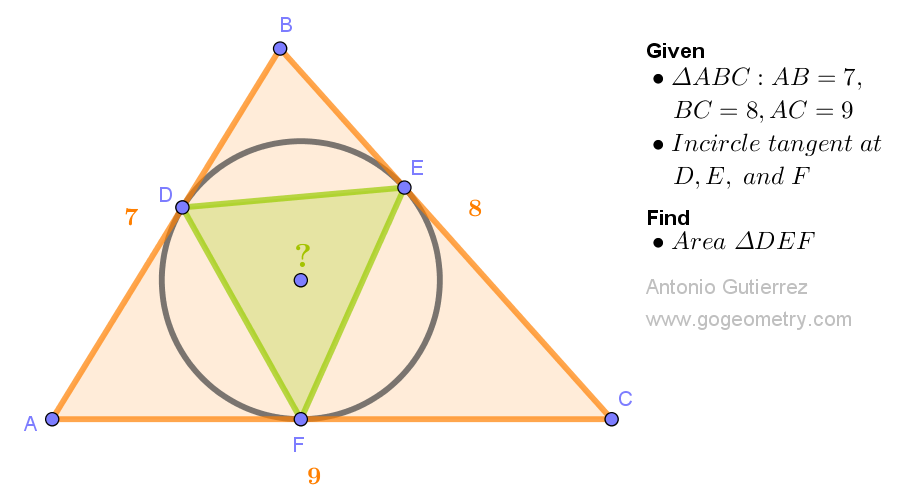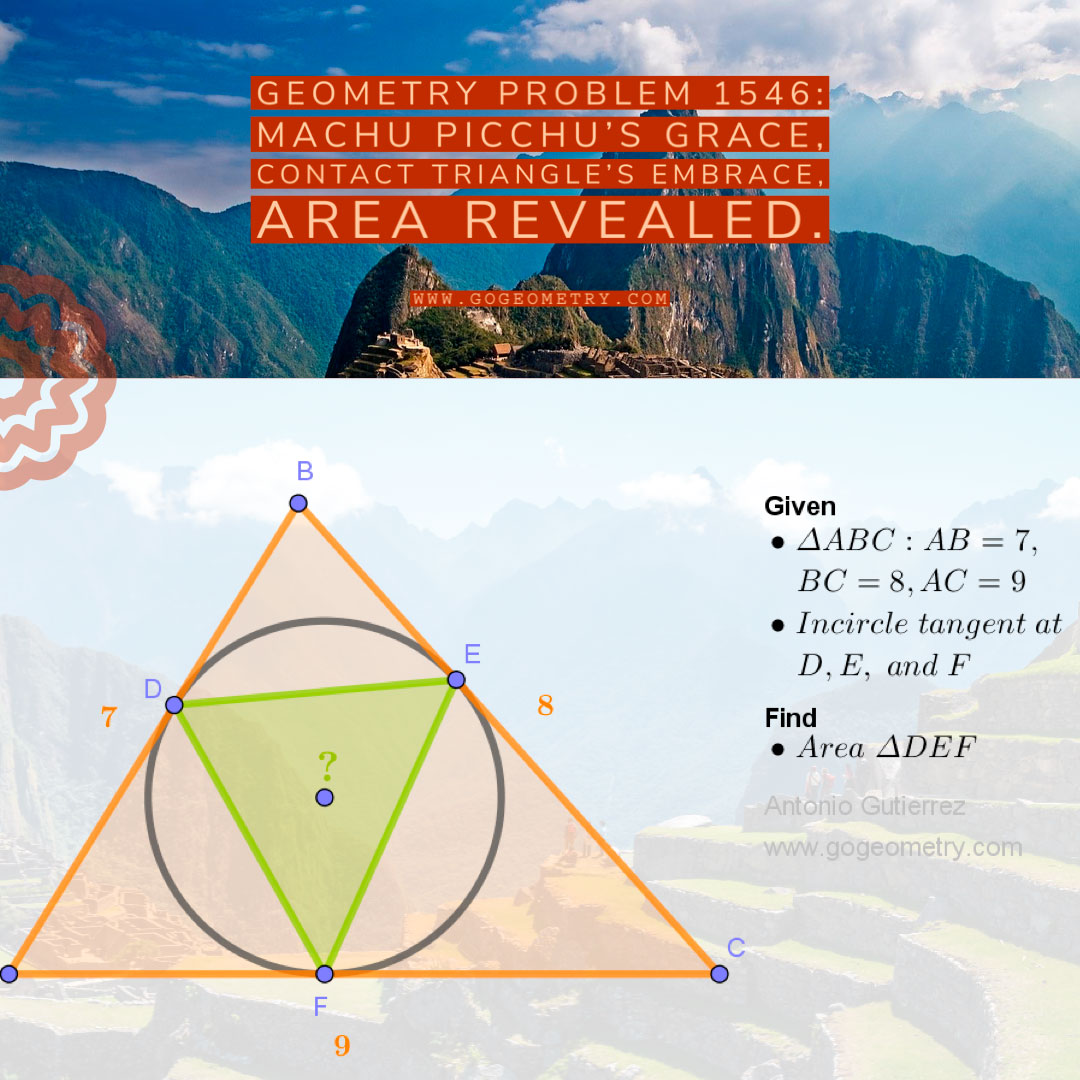# Geometry Problem 1546: Discover the Hidden Geometry: Calculate Area of Contact Triangle DEF in Triangle ABC

In triangle ABC, with side lengths AB = 7, BC = 8, and AC = 9, an inscribed circle is tangent to the sides AB, BC, and AC at points D, E, and F, respectively. Your task is to calculate the area of the contact triangle DEF.Geometry's embrace,
Inscribed circle's gentle touch,
Triangle's secrets.
Calculate, unveil the realm,
Contact triangle's beauty held.

## Lines and Shadows: Exploring Problem 1546 with Machu Picchu as the Backdrop